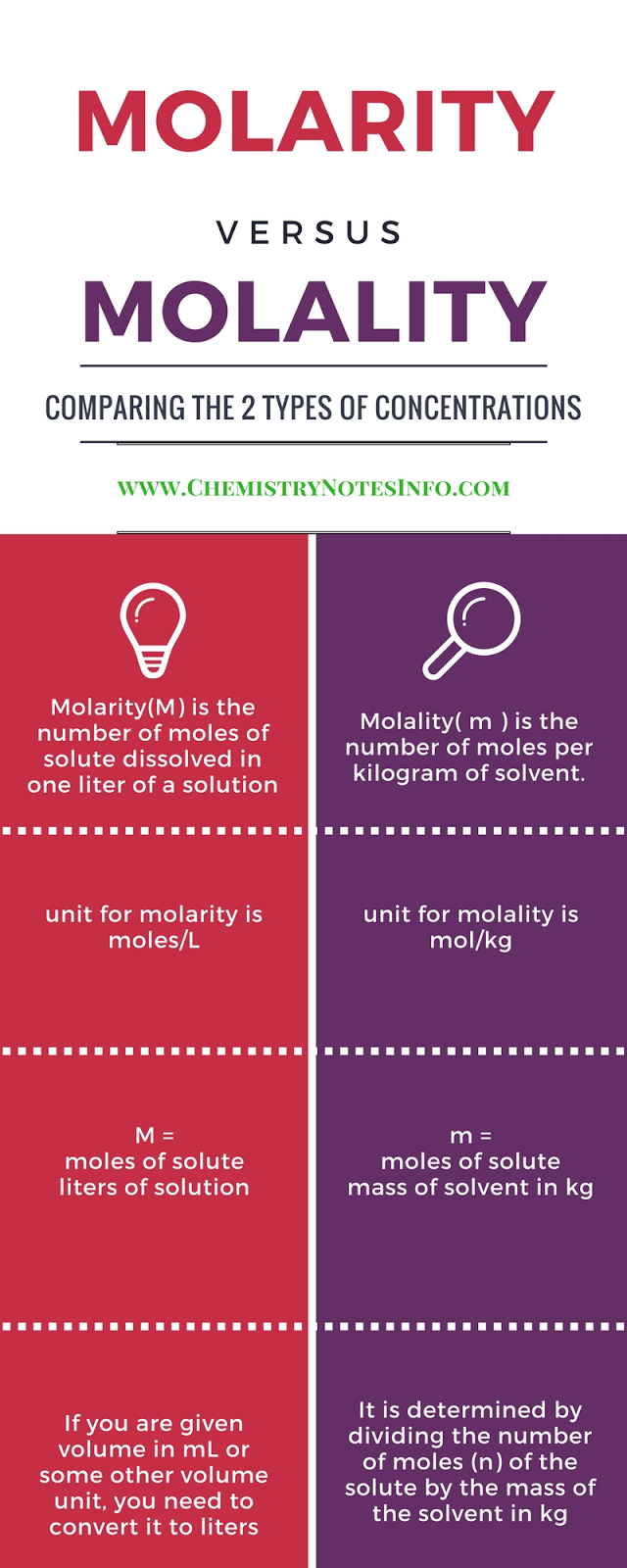# Molarity vs Normality

## What is Molarity ?

Molarity is also known as molar concentration, it is the ratio of moles of substance to volume in liter.
Where mole is weight in gram divided by molecular weight.
Molarity is chemistry terminology.

### Molarity (M) :-

No. of moles of the solute  / Volume of the solution in liter.

M = strength in gram per liter / Molar mass of the solute
Unit =>   M = Moles/L

## What is Normality ?

Normality is the ratio of gram equivalent weight to volume of solution in liter
where gram equivalent is the measure of reactive capacity of molecule consider for normality.

### Normality (N) :-

Gram equivalent weight  / Volume of the solution in liter.

Unit =>   N = "eq/L" (equivalent per liter)

Still have any doubt then view this Chemistry Info-graphics for
What is the difference between Molarity and Normality ?Molarity Vs Normality Chemistry Infographics

## What is Molality?

It is the ratio of moles of substance to kilogram of solvent.
Where mole is weight in gram divided by molecular weight.
Molality is chemistry terminology.

### Molality (m) :-

No. of moles of the solute  / Mass of solvent in Kilogram (Kg).

Unit =>   m = Moles/Kg

I think now you can easily differentiate between these terms means now you have answer of these two general questions i.e.
What is the difference between Molarity and Normality ?
What is the difference between Molarity and Molality ?

Still dont know then view this Chemistry Info-graphics for
What is the difference between Molarity and Molality ?Molarity vs Molality Chemistry Infographics